GRE Subject Test: Chemistry : Other Spectroscopy

Example Questions

Example Question #1 : Other Spectroscopy

Which formula gives the relationship between the energy (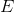) of a photon as a function of the frequency (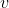) of light?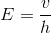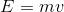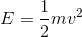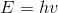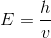Explanation:

There is a direct relationship between energy () of a photon and its frequency (). As energy increases the frequency associated with that photon increases based on the equation(=Planck's constant).

Example Question #21 : Spectroscopy

There are four laser pointers that give off different colors off light. The wavelengths corresponding to these colors are:

violet-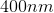red-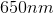green-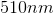blue-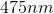yellow-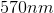Which laser pointer is expected to give off light with the highest energy?

Blue

Violet

Yellow

Red

Green

Violet

Explanation:

Violet waves have the most energy of the visible spectrum because of the relationship: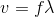Where=speed of light=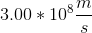,=frequency, and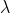=wavelength of light. The equation can be rearranged to:Therefore, there is an inverse relationship between wavelength and frequency. There is a direct relationship between energy and frequency governed by the equation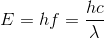Where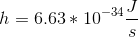is Planck's constant. So as frequency increases, so does the energy of the light waves. Therefore, waves with shorter wavelengths have higher frequencies, and more energy associated with them.

For the violet light: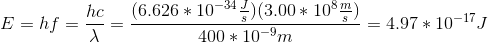If we complete this calculation for the other colors, our conceptual answer will be confirmed mathematically.

Example Question #22 : Spectroscopy

Which analytical method is used primarily to determine the functional groups present in a compound?

Nuclear magnetic resonance (NMR) spectroscopy

Ultraviolet-visible spectroscopy

More than one of these

Infrared (IR) spectroscopy

Mass spectrometry

Infrared (IR) spectroscopy

Explanation:

Infrared (IR) spectroscopy is used to determine the functional groups present in a molecule. It makes use of the IR region of the electromagnetic spectrum. When an analyte is exposed to IR radiation, different functional groups absorb radiation at their characteristic wavelengths causing vibrational and rotational excitation to bonds within the molecule. The frequencies absorbed correspond to the amount of energy required to increase the molecular vibration of the functional groups in a molecule and can be plotted on a graph for analysis. Mass spectroscopy is used primarily to determine the amount and types of chemicals present within a sample. Ultraviolet-visible spectroscopy is used to analyze highly conjugated compounds, biological molecules, and transition metals. NMR spectroscopy gives information about the electronic structure of a molecule.

Example Question #2 : Other Spectroscopy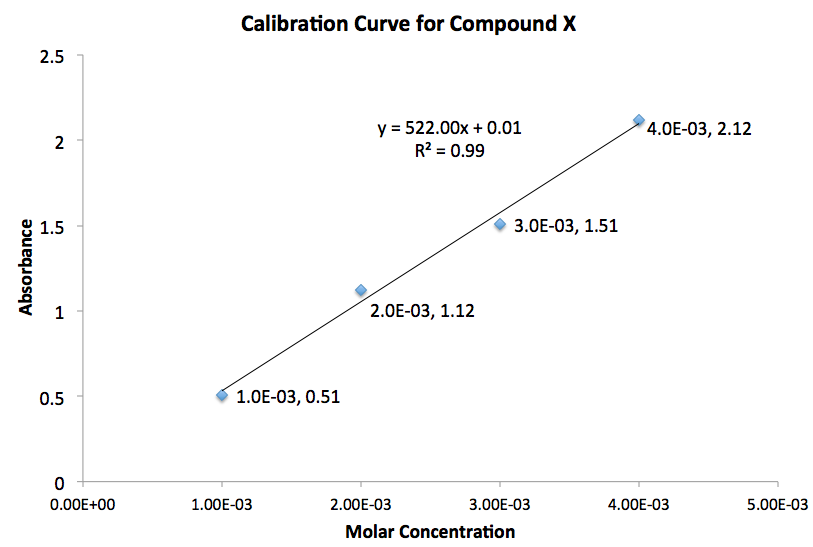In a UV-visible absorption spectroscopy experiment, the length of the cell or cuvette is 1cm. Given the calibration curve for a substance, what is the molar extinction coefficient for this compound?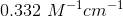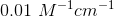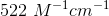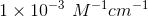Explanation:

The equation for Beer's law is: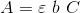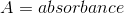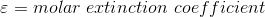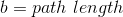Rearrange the equation: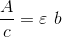Given that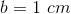, the slope of the line in the graph provided equals to the molar extinction coefficient. Therefore, the answer isAll GRE Subject Test: Chemistry Resources